## 1 Introduction

Heinz Lettau (1909–2005) was Professor of Meteorology at the University of Wisconsin from 1957 to 1980. He is considered the founder of the term planetary boundary layer (Sutton 1953), first published in Lettau (1939). Most of his publications deal with the atmospheric (planetary) boundary layer. For example, he was able to determine its essential properties by reworking the so-called Leipzig wind profile of Mildner (1932), as described in Lettau (1950). His contributions to atmospheric surface-layer research are relatively small, although, with the O'Neill experiment in 1953, he made a fundamental contribution to experimental boundary-layer research involving the layers near the ground (Lettau and Davidson 1957; Lettau 1990). In preparing this experiment, two years after moving from Germany to the USA in 1947 (Lettau 1990), he published a compilation of the state of knowledge on the atmospheric surface layer (Lettau 1949). In this work, a parameter y is inserted relatively abruptly that closely corresponds to the Obukhov stability parameter z/L (where z is the height and L is the Obukhov length) which is commonly used today (Fig. 1). As shown here, Lettau never explicitly stated the Obukhov length L and its meaning. This raises the question of whether Lettau developed this parameter himself or if he had access to Obukhov (1946; English translation Obukhov 1971) through his connections in Germany, in particular to the Central Meteorological Observatory in Potsdam, which was located after World War II in the Soviet occupation zone. This fundamental work of Alexander Mikhailovich Obukhov (see Yaglom 1990) was originally written in 1943, see Businger and Yaglom (1971). The above question, which is examined below, also requires insights into the life of Heinz Lettau in the period before, during, and after the war.

## 2 The Life of Heinz Lettau, with a Focus on the 1930s and 1940s

Heinz Helmut Max Lettau was born on 4 November 1909 in Königsberg, East Prussia (today Kaliningrad, Russia) into a merchant family. After completing a broad education in mathematics, physics, and geophysics, including meteorology, at the universities of Königsberg, Frankfurt am Main, and Leipzig, he received his doctorate in 1931 at the Geophysical Institute of the University of Leipzig (Weickmann and Börngen 2013) under Ludwig Weickmann (1882–1961) with a thesis on the 36-day air pressure wave. At this institute he met Katharina Dörffel (1910–2008), who also received her academic training at the Geophysical Institute, and they married in 1937. After a short period at the Geodetic Institute in Potsdam, he was Weickmann's assistant from 1933 to 1938, during which his workplace was at the Geophysical Observatory Collm. During this time, he wrote his habilitation thesis on the horizontal double pendulum (Lettau 1937) and his well-known book Atmospheric Turbulence (published in Leipzig in 1939). In 1938, he was appointed head of the Geophysical Observatory Gross Raum (today Rjabinowka) at the University of Königsberg and lectured in geophysics and meteorology.

After the outbreak of the Second World War, he was assigned to the Central Weather Service Group (ZWG), the main weather analysis and forecasting centre of the German Air Force, located in Wildpark near Potsdam, along with other outstanding meteorologists. Heads of the ZWG were Kurt Diesing (1885–1943) and, after his death, from August 1943 Werner Schwerdtfeger (1909–1985). In 1943, Lettau was offered a professorship at the University of Graz (Austria), but was unable to occupy the post, and the appointment was to be later revoked by the governor of the state of Styria after the end of the war. In the same year (1943), he was transferred to an air brigade under Colonel Max Wachtel (1897–1982). He was tasked with providing ballistic winds based on wind-profile and turbulence data for V-1 rocket launches, first at the Air Force test facility in Peenemünde and from spring 1944 in northern France. During an inspection trip to Rennes, he was taken prisoner of war by the Americans on 3 August 1944. After his release, he worked until 1947—again under Weickmann—as head of the scientific department at the Weather Service in the US zone in Bad Kissingen, before emigrating to the USA. He worked there at the Massachusetts Institute of Technology (Cambridge) and from 1957 to 1980 at the University of Wisconsin–Madison. He supervised numerous doctoral (more than 20) and master's theses (almost 30), and authored nearly 180 publications (a few are mentioned in the references).

Lettau was honoured both in the USA (becoming Fellow of the American Meteorological Society and receiving the Carl-Gustav Rossby Research Medal) and in Germany (Alfred Wegener Medal of the German Meteorological Society). He was a member of the Editorial Boards of Gerlands Beiträge zur Geophysik (until 1944) and Boundary-Layer Meteorology after its foundation in 1970. Heinz Lettau died on 3 August 2005 in Mount Pleasant, South Carolina. (Sources: Archive of the Leipzig University—UAL; Poggendorf 1959, 2003; Schwerdtfeger and Lettau 1986; Lettau 2004; Keller et al. 2008).

As suggested below, Lettau must have been aware of a few details of Obukhov's (1946) work as former colleagues from the ZWG or those in Potsdam and Leipzig (both in the Soviet sector of Germany) may have informed him. Before Lettau emigrated to the USA, from 1946 until the German currency reform in June 1948, contacts between the Western sectors and the Soviet sector in Germany were also quite possible. However, the exact manner in which this could have occurred is pure speculation.

## 3 State of Surface-Layer Theory at the End of the 1940s

Knowledge in micrometeorology was quite considerable at the time Obukhov (1946) und Lettau (1949) were published. The phenomenological understanding of the micrometeorological processes was especially well developed (Geiger 1927) as well as the understanding of the influence of plant-covered surfaces (Paeschke 1937). Also, the first heat balance of the Earth was available (Albrecht 1940) along with the first ideas of vortex structures near the Earth's surface (Albrecht 1943).

The theory of exchange processes was developed based on hydrodynamic considerations. The separation of mean motions and turbulent fluctuations had already been known for a long time as Reynolds decomposition (Reynolds 1895), while considerable progress was made with the definition of the Richardson number (Richardson 1920) and Prandtl's mixing-length approach (Prandtl 1925). The history of these findings is documented in Davidson et al. (2011). Relevant for their application in meteorology was the introduction of the "Austausch" (exchange) coefficient A by Schmidt (1925),

$$\tau = A\frac{\partial u}{\partial z} = \rho K \frac{\partial u}{\partial z} ,$$
(1)

with the turbulent stress τ, the air density ρ, the mean wind speed u, and the turbulent diffusion coefficient K.

This was an assumption for the atmosphere that followed the laws of hydrodynamics. At the same time, it was clear that the exchange coefficient had to be subject to strong temporal variations. Lettau (1939) defined an “apparent” exchange coefficient depending on the mixing length or the surface roughness. In Lettau (1941), he tried to vary the exchange coefficient in the diurnal cycle using periodic functions. Furthermore, a second maximum of the exchange coefficient at night was discussed (Lettau 1942; Robitzsch 1942), an effect that is nowadays found to be related to breaking gravity waves and low-level jets. The disadvantage of all these attempts was the lack of suitable observations.

Two problems of that time are specifically extensively quoted: the determination of the von Kármán constant κ and possible deviations from the logarithmic shape in velocity and temperature profiles. In relation to the first problem, Montgomery (1943, 1948) proposed a value of κ = 0.45 for the atmosphere in contrast to previous suggestions of 0.38 or 0.40. For the second problem, a dependence of the turbulent fluxes on stratification was found by Rossby and Montgomery (1935), and applied by Sverdrup (1936) as described in Sect. 4.1. Both Lettau (1949) and Obukhov (1946) made a reference to Sverdrup (1936), and Lettau (1939) made a reference to both Rossby and Montgomery (1935) and Sverdrup (1936). Most of the other papers did not mention these calculations; for example, Thornthwaite and Kaser (1943) found that, in the case of stable stratification, wind profiles no longer follow the logarithmic law. Sheppard (1947) found similar effects, with a part of the wind profile closest to the ground still following the logarithmic law, as Montgomery (1947) discussed which is in agreement with the later published theoretical interpretation by Monin and Obukhov (1954).

Richardson (1920) estimated the applied mechanical work of the shear stresses and the energy loss occurring with the thermal lifting of air parcels and the working of parcels against each other, which can be described by the ratio (Prandtl 1932)

$$- \frac{{\frac{g}{\rho } \frac{\partial \rho }{{\partial z}}}}{{\left( {\frac{\partial u}{{\partial z}}} \right)^{2} }} ,$$
(2)

where g is the acceleration due to gravity, which resulted in the Richardson number,

$${Ri} = \frac{{\frac{g}{\theta } \frac{\partial \theta }{{\partial z}}}}{{\left( {\frac{\partial u}{{\partial z}}} \right)^{2} }} ,$$
(3)

with θ being the potential temperature. In addition to Richardson and Prandtl, Taylor and Goldstein also investigated the problem (Prandtl 1932). Lettau (1939) followed the formulation of Prandtl and showed Eq. 2 explicitly. However, in Lettau (1949), the basis of the Richardson number is no longer presented. In contrast, Obukhov (1946) quotes Goldstein's textbook (Goldstein 1938).

## 4 Lettau’s Contributions to Surface-Layer Theory

### 4.1 Lettau’s Definition of the Obukhov Stability Parameter

While Lettau (1939) adhered strictly to the nomenclature given by Prandtl and Richardson, the accessibility of the work under consideration here (Lettau 1949) is relatively difficult, since Lettau (1949) used primes for differentiation with respect to height and used the French accent grâve for turbulent fluctuations (while the present work follows the notation that is common today). Furthermore, Lettau’s definition for the covariance for non-isotropic turbulence (grey-shaded equations and the sign of fluxes and parameters are identical to the original reference)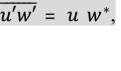(4)

is different from the other literature, where $$u^{*}$$ is referred to as a friction velocity, and $$w^{*}$$ as a mixing velocity, where $$u^{\prime }$$ and $$w^{\prime}$$ are turbulent fluctuations of the streamwise and vertical velocity components. According to the definition of isotropic turbulence (Taylor 1935), “In isotropic turbulence the average value of any function of the velocity components, defined in relation to a given set of axes, is unaltered if the axes of reference are rotated in any manner” and the associated assumption of an adiabatic atmosphere (subscript a) follows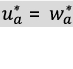and thus for the stress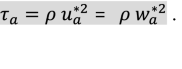(5)

Confusing in this context is the definition of the mixing length l with the local averaged vertical velocity component $$w^{*}$$ in Lewis (1997) about the balloon experiments of Lettau and Schwerdtfeger, for the time interval τ (not stress!)

$$l = w^{*} \tau = \mathop \int \limits_{t}^{t + \tau } w^{\prime } {\text{d}}t.$$
(6)

Lettau and Schwerdtfeger (1933a, b) used this approach to calculate the exchange coefficients by Hesselberg (1929) and Ertel (1932). However, they used the symbol $$\zeta^{*}$$ and not $$w^{*}$$. The symbol and the definition differ. In Lettau (1949), there is no reference to it.

For non-isotropic conditions, Lettau assumed that the ratio of mixing velocity and mixing length does not depend on the stratification(7)

The difference between adiabatic conditions and conditions with existing stratification is described by the buoyancy force. The term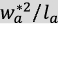describes the vertical component of turbulent acceleration under adiabatic conditions. Thus follows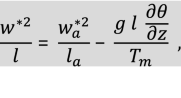(8)

with Tm as absolute mean temperature in the surface layer. From Eqs. 7 and 8, it follows that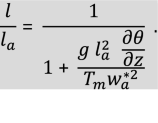(9)

Lettau used the notation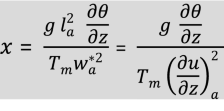(10)

and defined relationships between adiabatic and non-adiabatic conditions, for instance for the mixing length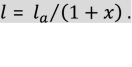(11)

He provided analogous relationships for the mixing velocity, the exchange coefficient (A, see Eq. 1), and the sensible heat flux (for which he used the symbol L instead of H in the following)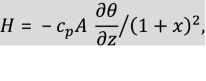(12)

where cp is the specific heat at constant pressure. Besides that, he related x to the Richardson number as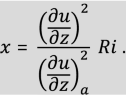(13)

At this point, Lettau introduced another dimensionless function of height, the adiabatic mixing velocity, and the sensible heat flux without further explanation (with the roughness length z0, see Fig. 1)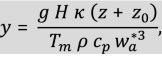(14)

which is nearly identical to the Obukhov stability parameter z/L defined by Eq. 19 below. For adiabatic and isotropic conditions, $$w_{a}^{*}$$ according to Eq. 5—see also Eq. 26a in Lettau (1949)—is equivalent to the friction velocity in today’s definition. From Eqs. 11 and 12 follows the relation between x and y,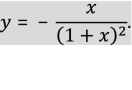(15)

Rossby and Montgomery (1935) used a similar approach to derive an equation for the vertical wind-speed profile, which means that they separated the adiabatic part from the stratification-influenced part. However, they did not separate the covariance $$\overline{{u^{\prime } w^{\prime } }}$$ according to Eq. 4. Finally, they found the relationship

$$\frac{{{\text{d}}u}}{{{\text{d}}z}} = \frac{1}{{\kappa \left( {z + z_{0} } \right)}}\sqrt {\frac{\tau }{\rho }} \sqrt {\frac{1}{2} + \frac{1}{2}\sqrt {1 + \frac{{4x^{2 } \left( {z + z_{0} } \right)^{2} }}{{\left( {\frac{1}{\kappa }\sqrt {\frac{\tau }{\rho }} } \right)^{2} }}} } .$$
(16)

With the definition by Rossby and Montgomery (1935)

$$x^{2} = \beta \frac{g}{T} \frac{{{\text{d}}\theta }}{{{\text{d}}z}} = \beta \frac{g}{T} \frac{H}{{c_{p} \rho \kappa u_{*} \left( {z + z_{0} } \right)}} ,$$
(17)

where β is an unknown dimensionless coefficient, it follows that

$$\frac{{4x^{2 } \left( {z + z_{0} } \right)^{2} }}{{\left( {\frac{1}{\kappa }\sqrt {\frac{\tau }{\rho }} } \right)^{2} }} = 4 \beta \kappa \left( {z + z_{0} } \right) \frac{g}{T } \frac{{\frac{H}{{c_{p } \rho }}}}{{u_{*}^{3} }} \approx 4 \beta \kappa z \frac{g}{T } \frac{{\frac{H}{{c_{p } \rho }}}}{{u_{*}^{3} }} = 4 \beta \frac{z}{L} .$$
(18)

Rossby and Montgomery (1935) used Eq. 16 to calculate the deviation of the non-adiabatic wind profile from the logarithmic wind profile for nearly isothermal conditions, and Sverdrup (1936) did the same for non-isothermal conditions. But they did not separate the term on the right-hand side of Eq. 18 and investigate its properties.

According to the current nomenclature (including the negative sign of L for unstable conditions with H > 0, no distinction between the friction velocity and the mixing velocity, and adiabatic conditions), from Eq. 14 with z » z0 and from Eq. 18 with β = 0.25, what follows is exactly the Obukhov stability parameter with the Obukhov length L

$$\frac{z}{L} = - \frac{{z \kappa \frac{g}{{T_{m} }} \frac{H}{{\rho c_{p} }}}}{{u_{*}^{3} }}.$$
(19)

However, this simplification is not in complete agreement with the mixing velocity according to Lettau (1949), because Eq. 5 assumes adiabatic conditions and isotropy, while in Eq. 19H ≠ 0 is possible. Businger (1955) already pointed out this problem in the discussion of Eq. 8. However, Obukhov (1946) and Monin and Obukhov (1954) give a range for z << L where the exchange conditions differ little from exchange conditions in a neutrally-stratified atmosphere independent of the magnitude of the sensible heat flux (sublayer of dynamic turbulence). Skeib (1980) (see also Foken and Skeib 1983) determined a critical height to which the assumption applies and which is 0.5 m to about 5 m depending on the stratification. Thus, it must be stated that the parameter y according to Lettau (1949) and z/L according to Obukhov (1946) are not exactly identical with respect to the definition, but are largely identical near the surface in the dynamical sublayer.

Monin and Obukhov (1954) also defined the Obukhov length as a function of the friction velocity, the sensible heat flux, and the buoyancy parameter $$g/T_{m} ,$$ but derived this relationship with the Π-theorem and not empirically. However, the relation between x and y or Ri and z/L was first obtained by Lettau (1949), earlier than Monin and Obukhov (1954), and is still used today with little numerical difference for stable stratification (Businger et al. 1971)

$${\raise0.7ex\hbox{z} \!\mathord{\left/ {\vphantom {z L}}\right.\kern-\nulldelimiterspace} \!\lower0.7ex\hbox{L}}\, = Ri\quad{\text{for}}\,Ri < 0,$$
(20)
$${\raise0.7ex\hbox{z} \!\mathord{\left/ {\vphantom {z L}}\right.\kern-\nulldelimiterspace} \!\lower0.7ex\hbox{L}} = \frac{Ri}{{1 - 5 Ri}} \quad{\text{for}}\,0 \le Ri \le 0.2 = Ri_{c} .$$
(21)

### 4.2 Obukhov’s Definition of the Obukhov Length

Obukhov (1946) also used Schmidt's exchange approach. Obukhov solved the problem of stratification by using a function of the Richardson number,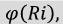where in the neutral case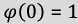. It is interesting that Obukhov also began his derivation with two generalized velocities, the friction velocity (symbols according to Obukhov 1946)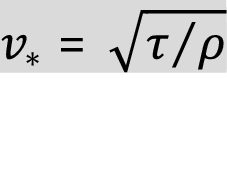(22)

and the "thermal" mixing velocity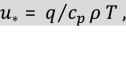(23)

with the sensible heat flux q. [Remark: "thermal" mixing velocity, in Russian: cкopocть пepeнoca тeплa; in the English translation (Obukhov 1971) a formulation according to current usage was chosen: “heat flux velocity” with the symbol u, in Russian: cкopocть пoтoкa тeплa]. This unusual use of $$u_{*}$$ may be confusing to the reader. Note that this also differs from Lettau’s definition of the mixing velocity $$w^{*}$$.

Obukhov did not make a distinction between isotropic and non-isotropic turbulence, and so for the Richardson number it follows that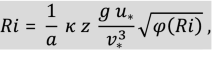(24)

where 1/a is the turbulent Prandtl number according to our present-day definition. The derivative with respect to height is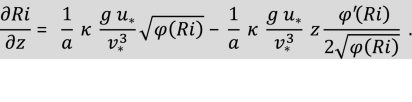(25)

The value of the derivative at z = 0 was defined by Obukhov as the scale of the height of the surface layer with dynamic turbulence(26)

with a = 1. For a discussion of the use of the turbulent Prandtl number and the von Kármán constant by different authors, see Foken (2006). The Obukhov length is significantly greater than the height of the surface layer for neutral stratification. A physical interpretation of L was made by Bernhardt (1995), i.e., “the absolute value of the Obukhov length is equal to the height of an air column in which the production (L < 0) or the loss (L > 0) of turbulence kinetic energy (TKE) by buoyancy forces is equal to the dynamic production of TKE per volume unit at any height z multiplied by z”.

### 4.3 Properties of the Obukhov Stability Parameter

In the early 1950s, Lettau was obviously quite convinced of his scaling velocity $$w_{a}^{*}$$. However, when results of the O'Neill experiment of 1953 were published in 1957, references to $$w_{a}^{*}$$ were already missing (Lettau 1957, 1990) and it was not adopted by the community (Sutton 1953). Lettau's ideas are partly contradictory to the scaling of Batchelor (1950). This was explained by Inoue (1952) in a commentary to which Lettau (1952a) replied and specified his ideas. In particular, they referred to the extension of the scaling into the laminar domain and the application of Taylor's (1935) definition of isotropic turbulence for the atmosphere. Lettau again presented his ideas in a broader context in Lettau (1952b).

It is appropriate to look again at Obukhov (1946) and Lettau (1949) with regard to the further explanations. Both authors show an almost identical picture with the dependence between Ri and z/L or x and y, respectively (Fig. 2). It is noticeable that the range of validity for z/L and y was chosen to be relatively broad (as Lettau showed in another figure, even for − 5 < y < 15 as well). In contrast, the range of validity of Monin and Obukhov's (1954) similarity theory for the surface layer today is given as − 1 < z/L < 1 when defining the Obukhov length with the von Kármán constant and without the turbulent Prandtl number (Foken 2006), whereby influences of the mixed layer and the Coriolis force are certainly present (Johansson et al. 2001; Högström et al. 2002).

It must therefore be assumed that, based on his earlier works, Lettau always had the entire boundary layer in view. It is thus not unreasonable to define a thermal mixing velocity. This idea was also used by Zilitinkevich (1971) and Betchov and Yaglom (1971) for the convective atmospheric boundary layer, summarized by Kader and Yaglom (1990), and can be seen as an anticipation of Deardorff's (1970) scaling velocity for convective conditions, even if it is defined differently as.

$$w_{*} = \left( {\frac{{g z_{i} }}{{\theta_{v} }} \overline{{w^{\prime } \theta_{v}^{\prime } }} } \right)^{1/3} ,$$
(27)

where $$z_{i}$$ is the mixed-layer height, and $$\theta_{v}$$ is the virtual potential temperature. Interesting is the choice of the symbol for the Deardorff velocity, which is still valid today, even if there is no explicit reference to Lettau in Deardorff’s work.

An essential application of the parameter y (or z/L) is as a stability-dependent term in the profile equations for the momentum or heat exchange. Obukhov (1946) as well as Lettau (1949) tried this by modifying the exchange coefficient. In the case of Lettau, the following equation of exchange coefficient—using Eq. 10—was formulated as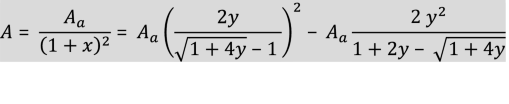(28)

because(29)

Physically, only the root of the smaller absolute value is of interest.

Thus, Lettau has explicitly introduced the parameter y (or z/L) into the profile equations, similarly to Rossby and Montgomery (1935) and Sverdrup (1936). However, the formulation led to considerable problems in integration, and he developed the root expression as a power series.

In contrast, Obukhov modified the exchange coefficient with a function depending on the ratio of Richardson number and critical Richardson number $${Ri}_{{{\text{cr}}}}$$.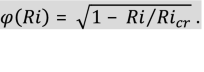(30)

In an intermediate step, Lettau formulated a parameter $${\raise0.7ex\hbox{{\beta z}} \!\mathord{\left/ {\vphantom {{\beta z} L}}\right.\kern-\nulldelimiterspace} \!\lower0.7ex\hbox{L}}$$. However, this parameter was first introduced into the profile equations by Monin and Obukhov (1954) as a power series $$1 - {\raise0.7ex\hbox{{\beta z}} \!\mathord{\left/ {\vphantom {{\beta z} L}}\right.\kern-\nulldelimiterspace} \!\lower0.7ex\hbox{L}}$$, the so-called universal function of the similarity theory of Monin and Obukhov.

Lettau (1956) used his formulation (Lettau 1952b) in comparison with observations and found a clearly better agreement than with the theory of Businger (1955), who preferred a purely modified mixing-length approach with references to Rossby and Montgomery (1935) and Lettau (1949). Therefore, Businger’s approach has similarities to that of Lettau (1949) and contrasts to the statistical turbulence approaches of Batchelor (1950) and Inoue (1952).

## 5 Conclusions

A first conclusion is that Rossby and Montgomery (1935) were the first to find a term in the non-adiabatic profile equation that was similar to the Obukhov stability parameter z/L and was applied by Sverdrup (1936). However, they did not explicitly discuss this parameter. Because Obukhov and Lettau were familiar with Sverdrup (1936), this term was not unknown to both.

On the one hand the following assessment is hypothetical as arguments cannot be dismissed that Lettau knew at least several results of Obukhov’s 1946 paper. On the other hand, Lettau's work of 1949 was certainly not completely unknown to Monin and Obuknov, see Monin and Obukhov (1954). A possible, albeit rather improbable, contact person in the years 1945 to 1948 could have been Prof. Hans Ertel, who from 1946 was Professor for Geophysics in Berlin (East), and Lettau used his exchange approach (1932). A further possible contact was Prof. Horst Philipps, with whom Lettau served, at least temporarily, within the Central Weather Service Group of the German Air Force during World War II. After 1946, Philipps was at the Meteorological Central Observatory Potsdam in the Soviet occupation zone. Philipps and Dr. Fritz Albrecht worked there on Soviet research projects (Möller 1963) investigating the heat balance of the Earth’s surface (Albrecht 1950). Albrecht supported Lettau’s balloon experiments with scientific devices (Lettau and Schwerdtfeger 1933b) and he used the Sverdrup (1936) approach for his own work (Albrecht 1940, 1943). In 1948, he emigrated to the western part of Germany and in 1949 for eight years to Australia, and later again back to Germany (Möller 1965; Foken and Spänkuch 1996).

It is also possible that the contents of Obukhov (1946), together with the publication of Kolmogorov's famous papers and Obukhov's supplementary paper (Kolmogorov 1941a, b; Obukhov 1941; Falkovich 2011), were brought to the English-speaking world by Batchelor 's (1947) contribution.

Obukhov's definition of two velocity scales is confusing, especially because one of these is the sensible heat flux normalized with the temperature (Eq. 23) and having the dimensions of a velocity. Lettau must have heard of this somehow and tried to define two scales of velocity, because he developed the “thermal” mixing velocity for w*. This took him as far as the definition of the parameter x (Richardson number) but not to the derivation of the parameter y, which he introduced rather speculatively, since he obviously knew about it.

In conclusion, it must be noted that Lettau never explicitly discussed the Obukhov length and thus had not recognized its universal character as Obukhov (1946) and Monin and Obukhov (1954). However, he was aware of the stability parameter z/L and the properties of this function, so the work of 1949 can certainly be understood as a link between Obukhov (1946) and Monin and Obukhov (1954). It is significant that Lettau also never claimed to have found the Obukhov length, up to the award of the Alfred Wegener Medal in Germany in 1974, when, in a reference to a lecture by Neumann (1964), he is described as the second inventor of the Obukhov length (Wippermann 1974). In the 1974 laudation for the Carl-Gustaf Rossby Research Medal of the American Meteorological Society "his original concept of the stability length scale” was highlighted (AMS 1974) as well. In 1979, Lettau (1979) first obviously pointed out, with reference to Neumann's lecture and Stearns (1970), that he had derived a term with a similar meaning but with a different scaling in 1949. At least since 1971 (Businger and Yaglom 1971) it has been known in the English-speaking world that the length scale was proposed as a scaling parameter by Obukhov in 1946. Also, Businger and Yaglom (1971) wrote: “This length scale was later introduced independently by Lettau (1949)”. Consequently, it can be said that Lettau investigated the fundamental properties of the stability parameter z/L and it would be appropriate to name z/L the Obukhov–Lettau stability parameter.